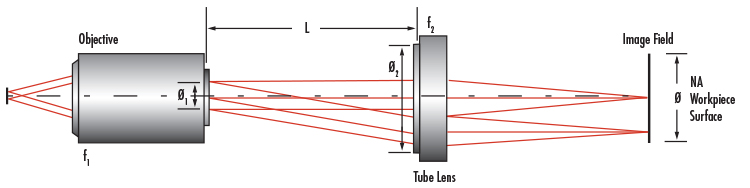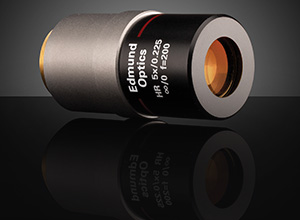# Infinite Conjugate Tube Length

View All Technical Tools

Objective Focal Length (mm):
Objective NA:
Tube Lens Entrance Pupil Diameter (mm):
Tube Lens Focal Length (mm):
Horizontal (mm):

Vertical (mm):

Diagonal (mm):

### --

Objective Exit Pupil Diameter (mm): --

Objective Tube Lens Distance (mm):--$$∅_1 = 2 \, f_1 \, \text{NA}$$
 $$L = \left( \frac{∅_2 - ∅_1}{∅} \right) f_2$$
 f1 Objective Focal Legnth f2 Tube Lens Focal Length ∅1 Objective Exit Pupil Diameter ∅2 Tube Lens Entrance Pupil Diameter
 ∅ Sensor Size NA Objective NA L Objective Tube Lens Distance

## Example

Question: Using an M Plan APO 10X objective (#46-144), MT-1 tube lens (#54-774), and a 2/3” sensor camera, what is the maximum spacing between the tube lens and objective without vignetting?
Answer: The focal length of the objective (f1) is 20mm and its NA is 0.28, so the exit pupil diameter can be calculated by:

$$∅_1 = 2 \times 20 \text{mm} \times 0.28 = 11.2 \text{mm}$$

A 2/3” image sensor features an 11mm diagonal, therefore ∅ needs to be at least 11mm. The focal length of the MT-1 tube lens is 200mm and the entrance pupil diameter is 24mm. Therefore,

$$L = \left( \frac{24 \text{mm} - 11.2 \text{mm}}{11 \text{mm}} \right) 200 \text{mm} = 232.7 \text{mm}$$

As long as the spacing between the tube lens and objective is less than 232.7mm, there will be no vignetting.

## Description

Determine the maximum tube length in an infinite conjugate microscope system for covering a specific image sensor size without vignetting using the objective focal length, objective NA, tube lens entrance pupil diameter, tube lens focal length, and sensor size. The distance between the objective and the tube lens in an infinity corrected microscope can be varied, but this will affect the image field diameter. Using a tube length less than this maximum value will prevent vignetting.

## Related Resources and Products